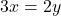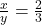Elena has a shop on Etsy where she makes and sells belts and headbands for girls out of fancy ribbons. Each set uses a total of 1 yard (36 i

Question

Elena has a shop on Etsy where she makes and sells belts and headbands for girls out of fancy ribbons. Each set uses a total of 1 yard (36 inches) of ribbon. She cuts this into two pieces so that the length of the first piece divided by the length of the second piece has a ratio of 2 to 3.

in progress 0
5 months 2021-08-11T11:15:13+00:00 1 Answers 2 views 0

1. Missing part of the question:

Let  x represent the length of the first piece. Write an equation that represents the ratio of the first piece of ribbon to the second piece. Be sure to define your variable or variable expressions.Step-by-step explanation:

Given

x = first

y = second

Required

Represent as an equation

From the question, when x is divided by y, it gives ratio of 2 to 3.

This implies that:Cross Multiply